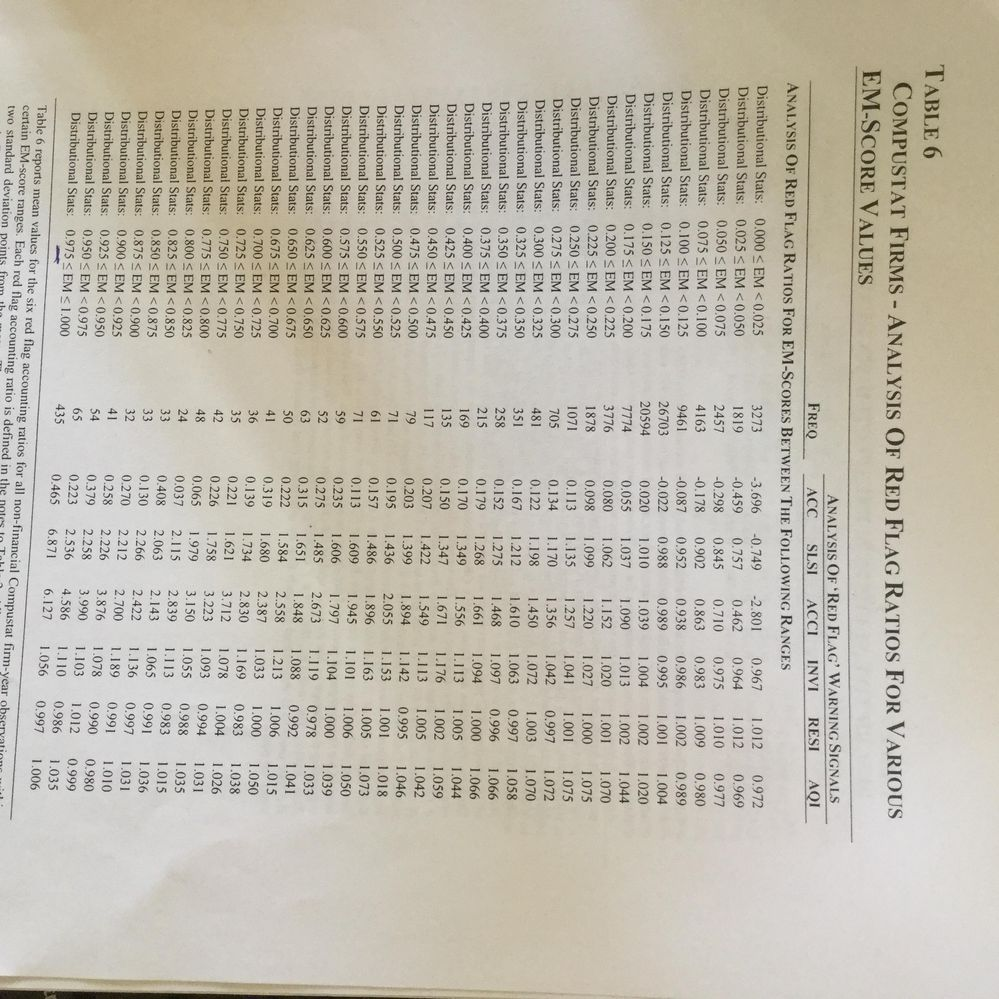## Summary statistics by range

Hi SAS community,

i have a variable called em. It range from 0 to 1.

i would like to create a summary statistics table for other variables based on the ranges of em.

for example, I would like to get the mean of variables when the observation has 0<=em<0.025, 0.025<=em<0.050 ect.

many thnaks.

1 ACCEPTED SOLUTION

Accepted Solutions

## Re: Summary statistics by range

1. Create categories for EM. Either create a new variable and recode it to the values you want or create a format and apply it to the variable.

2. Use Proc means, include your new variable from 1 as a Class variable, or the EM variable if you used a format.

Proc means data=have mean nway;

class em;

format EM em_cat.;

var var1 var2 var3;

output out = want mean= /autoname;

run;

4 REPLIES 4

## Re: Summary statistics by range

To make it clear, I would like to get a table like this one.

thank you very much## Re: Summary statistics by range

1. Create categories for EM. Either create a new variable and recode it to the values you want or create a format and apply it to the variable.

2. Use Proc means, include your new variable from 1 as a Class variable, or the EM variable if you used a format.

Proc means data=have mean nway;

class em;

format EM em_cat.;

var var1 var2 var3;

output out = want mean= /autoname;

run;

## Re: Summary statistics by range

Thank you Rezza, but how I can create the categories for em?

## Re: Summary statistics by range

Use if then else in a data step.

http://www.ats.ucla.edu/stat/sas/modules/vars.htm

See example 2.

Discussion stats
• 4 replies
• 1301 views
• 2 likes
• 2 in conversation